Historical Archive of the Bible Wheel Site

The Bible Wheel had been debunked by its author.
Read all about it: Debunking Myself: What A Long Strange Trip It's Been

Recent Blog ArticlesAnd for that the dream was doubled unto Pharaoh twice; it is because the thing is established by God, and God will shortly bring it to pass.

Genesis 41:32

The term repdigit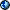denotes any integer that is represented by single repeated digit, such as 11, 666, 777777, or 9999999. Any repdigit is a multiple of a repunit. For example, the repdigit 999 is equal to 9 times the repunit 111. The repunits are denoted by Rn, where n indicates the number of repeated units. For example: R3 = 111 and R4 = 1111. Thus we write 999 = 9 x R3. When confusion is unlikely, the multiplication sign can be dropped, and we will write 999 = 9R3.

The value of a repunit depends on the base in which is it written because it is defined in terms of its digits. For example, the number 7 in base 10 is a repunit in base 2, because it is represented by writing 111 in that base. In general, repunits in base b are given by the following formula:

Rn = (bn - 1)/(b - 1)

For example, in base 10 we have b = 10 and R3 = 111:

R3 = (103 - 1)/(10 - 1) = 999/9 = 111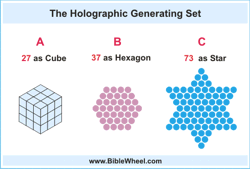I became fascinated with repunits and repdigits after discovering that they are fundamentally related to the Holographic Generating Set (GenSet for short) derived from the alphanumeric integration of the introductory verses of Genesis and John (see Creation Holograph). The GenSet is defined as the set (A = 27, B = 37, C = 73). These three numbers are geometrically related, consisting of the third Cube, 33 = 27, and the fourth Hexagon/Star pair 37/73. These number forms are closely related because Hexagons give the differences between successive Cubes. For example:

43 - 33 = 64 - 27 = 37 = Hex(4)

When the GenSet is augmented to include the sum of its elements, D = A + B + C = 137, we find the famous first aproximation to the Fine Structure Constant which governs the interaction of Light and Matter, a subject intimately related to the creation passages in Genesis and John. The Creation Holograph reveals an amazingly dense set of self-reflective alphanumeric and geometric relations that can be expressed as simple combinations of GenSet elements. Here are a few of the most obvious (recall Tri(n) denotes the nth triangular number):

Genesis 1:1 = 100A + 1 = BC = Tri(C) = Tri(B) + 2AB = 100B - AB

Genesis 1:1 + AB = John 1:1 + C = AD + 1 = 100B

Genesis 1:1 + John 1:1 = AB + C2 = Tri(112)

The first line shows five independent representations of Genesis 1:1, with two of them based on triangular forms of GenSet numbers, which are themselves geometric. Multiples of 10 and 100 appear frequently. Besides its integration with the Creation Holograph (from which it was derived), the GenSet is also extremely self-reflective and deeply integrated with base 10, as seen in this table of binary products:

 Selected Binary Products from Generating Set BC 37 x 73 2701 2700 + 1 A x 102 + 1 AD 27 x 137 3699 3700 - 1 B x 102 - 1 AA 27 x 27 729 730 - 1 C x 101 - 1 BB 37 x 37 1369 1370  - 1 D x 101 - 1 CD 73 x 137 10001 10000 + 1 ....104 + 1 AB 27 x 37 999 1000  - 1 ....103  - 1

The table shows the relation between all four elements of the GenSet with themselves and factors of 10, 100, 1000, and 10000 plus or minus one. These identities, and many more, are discussed in the GenSet article. Of utmost significance is its relation to the name of our Creator: Jesus Christ = 2368 = AB + B2 = Cube(4) x Hex(4).

To see the connection between the GenSet and the repdigits, we begin by considering repdigits of the form 9R2m. These repdigits can be algebraically factored as follows:

9R2m = 102m - 1 = (10m - 1)(10m + 1)

This is the standard formula for the difference of squares which can always be algebraically factored as x2 - y2 = (x - y)(x + y). This means that when n is even, the factors of 9Rn follow a symmetric pattern:

 9R2 = 99 = 9 x 11 = (101 - 1)(101 + 1) 9R4 = 9999 = 99 x 101 = (102 - 1)(102 + 1) 9R6 = 999999 = 999 x 1001 = (103 - 1)(103 + 1) 9R8 = 99999999 = 9999 x 10001 = (104 - 1)(104 + 1)

The first thing to note is that 9R2m = 9Rm x (10m + 1). For example, 9R6 = 9R3 x 1001. Thus, the even repdigits are just multiples of the odd repdigits. This is a special case of the theorem that says "If n is a multiple of m, then Rn is a multiple of Rm". Using the mathematical notation of a vertical line to signify the word "divides", this theorem is written "If m|n then Rm|Rn" and read as "If m divides n, then Rm divides Rn."

The connection with the GenSet is now obvious. All four elements of the augmented GenSet are found as paired factors of the repdigits 9R3 and 9R8:

 From 9R3: 999 = 103 - 1 = x    = AB From 9R8: 10001 = 104 + 1 = x = CD

The pattern of powers of ten plus or minus one recalls what we saw in the table of binary products above. This is the wonder of the GenSet. It was designed by God to lead us ever deeper into His mathematical design of all creation. I originally derived it from the Creation Holograph. I then discovered that it could be defined from the continued fraction expansion of the Fine Structure Constant (which itself forms a profound connection between Quantum Physics and the alphanumeric structure of Scripture). And now I see that it relates to the repdigits.

Another link between the Repdigits and the Creation Holograph is found in the prime number which divides R5 = 11111 = 41 x 271. Like the elements of the GenSet, this number is geometric. It is the Tenth Centered Hexagon Number, 271 = Hex(10) = 103 - 93. It plays a very significant role in the creation of light fragment of the Creation Holograph, where it is symmetrically coordinated with the Number 37 = B = Hex(4):These symmetrically coordinated numbers from the Creation Holograph are also symmetrically coordinated factors in the 3rd and 5th repdigits:

 9R3 = 999 = 27 x 37 = AB 9R5 = 99999 = 271 x 369 = (10A + 1)(10B - 1)

There are many repetitive patterns with variations on themes, such as the formal similarity to the factors of even repunits R2m = (10m + 1)(10m - 1) and the binary products of GenSet elements. Another variation is on the theme of "2 and 7" in the numbers , 207, , which all play essential roles in the Creation Holograph. And yet another fascinating variation is seen in the table of the GenSet reciprocals:

 Reciprocals of Generating Set Elements 1/A = 1/27 = 0.037037037037 ... repeating digits of B 1/B = 1/37 = 0.027027027027 ... repeating digits of A 1/C = 1/73 = 0.01369863... repeating digits of 1369863 = 9999D and 1369 = B2 1/D = 1/137 = 0.00729927... repeating digits of 729927 = 9999C and 729 = A2

The digits in the GenSet reciprocals are multiples of the GenSet elements, just as we saw with their binary products. The GenSet is profoundly self-reflective and integrated with the repdigits. It has unique properties found in no other set of numbers, especially in light of their additional geometric nature. It is truly amazing that this set was discovered through the analysis of the intrinsic alaphanumeric structure of the creation passages of the Holy Bible. What a witness to the wisdom of our Creator and Teacher! So now the big question is this - "What is the mathematical and symbolic significance of the repdigits?" The answer is no less astounding than everything that led up to the question. As it turns out, the repdigits are fundamental to the restoration of unity following the division that was required for creation.

### Repdigits, Creation, and the Restoration of Unity following Division

In the beginning God created the heaven and the earth. And God said, Let there be light: and there was light. And God saw the light, that it was good: and God divided the light from the darkness.

Genesis 1:1-4

How does God create the universe? The Bible reveals that He created all things through His Word, as it is written:

In the beginning was the Word, and the Word was with God, and the Word was God. The same was in the beginning with God. All things were made by him; and without him was not any thing made that was made. In him was life; and the life was the light of men. And the light shineth in darkness; and the darkness comprehended it not.

John 1:1-5

The word translated as "Word" in John 1:1 is Logos. This also is a title of the Second Person of the Godhead, "whose name is called the Word of God" (Rev 19:13). This is the deepest meaning of the Second Letter (Bet = House), which simultaneously signifies the Son of God (Bet KeyWord Ben, see Second Psalm) and the Word of God (see Logos Holograph = Hebrews 4:12 and Bet = 412 = 2 x Word (Davar)). All these ideas are ultimately subsumed under the Numerical Category defined by the Number 2, which contains all concepts relating to Division, Duality, Image, and Reflection. Thus the Son (Ben) is the Second Person of the Trinity and the "image of the invisible God" (Col 1:15). The Number 2 is one of the most obvious symbolic numbers in Scripture. Its meaning can be traced very easily through the Second Day (Division of the Waters), the Second Commandment (No graven images), the Second Seal (Great Sword = Instrument of Division). Ultimately, it is based on the meaning of the name of the Second Letter (Bet = House) as that which divides between what is "in" and what is "out" and this is basis of the very concept of "definition" and so lies at the root of the meaning of "the Word" that divides between what "is" an "is not" - the very Sword of the Spirit by which God created all that is (Ephesians 6:17). This is the "sharp two-edge sword" emerging from the mouth of our Creator, the Lord Jesus Christ (Rev 1:16). Thus we see that the biblical revelation of how God created, including the essence of the concept of "word" itself, is based on the nature of the Triune Godhead and embedded in the symbolic strucutre of the Hebrew alphabet! Endless glory! The Christian revelation is the true revelation of Ultimate Reality! This is explained in great detail in the Synopsis of Spoke 2 in the Bible Wheel book.

The manifold connections between the Number 2 and the concepts of Division, Word, and the process of Creation form a very dense symbolic matrix that unites the Bible Wheel, Biblical Holographs, and Trinitarian Theology. The intuition that there also was some deep connection with the repdigits has been floating in the back of my mind for many years now. The full understanding finally emerged when I looked closely at what happens when unity is divided. Consider what happens when we divide unity by seven:

1/7 = 0.142857 142857 142857 142857 142857 ...

The repeating sequence of digits - 142857 - is called the repetend, which I separated with spaces for clarity. All such repetends are factors of the repdigits because the fraction must be returned to unity when multiplied by its divisor:

1 = 7 x (1/7) = 7 x 0.142857 142857 142857... = 0.999999 999999 999999 ...

Many folks are surprised when they first learn that an infinite sequence of nines following the decimal point is exactly equal to unity. But a little reflection on what happens when we divide and multiply should make it clear. Consider 1/3 = 0.333333.... When we multiply by 3 we must arrive at 1 = 3 x (1/3) = .999999.... This is why the repetends are always factors of the redigits:

7 x 142857 = 999999 = 106 - 1 = 9R6

Note that all the numbers in this equation are integers, and that the divisor is related to the exponent of the number 10: 6 = 7 - 1. The number 7 is called a Full Repetend Primein base 10 because its repetend consists of six digits, the maximum possible for the number 7. This maximum is found using Fermat's Little Theorem:

ap-1 = 1 mod p if p does not divide a.

This theorem shows that p will always divide 10p-1 - 1 = 9Rp-1 for all primes p other than 2 or 5 which are excluded because they divide 10. Fermat's Little theorem gives the largest possible value of a period length for a prime. The exact value is given by Euler's Totient Function, phi(p). Here is a table of the first four Full Repetend Primes that shows how they relate to the repdigits:=7 x 142857 = 9R6=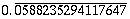17 x 588235294117647 = 9R16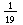=19 x 52631578947368421 = 9R18=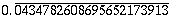23 x 434782608695652173913 = 9R221 x 142857 = 142857
3 x 142857 = 428571
2 x 142857 = 285714
6 x 142857 = 857142
4 x 142857 = 571428
5 x 142857 = 714285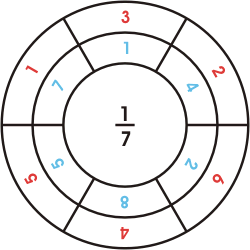1 + 6 = 7 1 + 8 = 9 3 + 4 = 7 4 + 5 = 9 2 + 2 = 7 2 + 7 = 9

The Full Repetend Primes generate the cyclic numberswhich got there name from the fact that their digits cycle through the various possible positions while remaining in the same order. The first digit moves to the last position, the second to the first, and so on, as shown for the cyclic number generated by the reciprocal of seven. I have shown the long division on the right using blue for the sequence of quotients and red for the sequence of remainders. The facinating thing about cyclic numbers is that the sequence of remainders 1 3 2 6 4 5 gives the sequence of multiples that shift the digits one space over. The circle is a convenient way to display patterns found in the repeating digits. The inner circle shows the quotient in blue, and the outer circle shows the remainder in red. The circles have interesting patterns and symmetry. Starting at the top of the inner circle and reading clockwise, we find the digits of the repetend, 142857. Starting once space back from the top of the outer circle gives the sequence of remainders. Remainders (red) that lie on opposite sides of the circle always add to the value of the divisor (7), and quotients (blue) that lie on opposite sides always and to one less than the base (9), which is the digit needed to return to unity. To find where the sequence starts for a given multiple, look for the corresponding remainder and begin with the blue digits one space clockwise. For example, to find the sequence for 6 x 142857, start with blue eight on the inner circle clockwise from the red 6 on the outer circle and read clockwise to get 857142.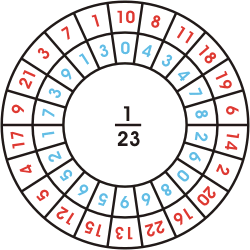Wheel of 22 Spokes generatedby the Number 23 = Aleph + Tav

Number 23, the fourth Full Repetend Prime, generates a wheel of 22 Spokes just like the Bible Wheel. The same patterns of opposite remainders (red) adding to the divisor (23) and opposite quotients adding to 9 is valid for all Full Repetend Primes. Not all primes are Full Repetend Primes. The sequence begins 7, 17, 19, 23, and may be viewed here. I find it intriguing that the Bible Wheel corresponds to the cycle of length 22 generated by the fourth Full Repetend Prime, the Number 23, which is the ordinal value of Aleph (1) + Tav (22), adn that these letters combine to seal the Wheel with the word(ot) meaning divine sign, miracle, proof, or signature. My intuition now is leading me to think there is a fundamental link that extends form the Creation Holograph and the GenSet through the Repdigits and Cyclic Numbers all the way to the large-scale structure of God's Word in the form of the Wheel. Facinating stuff! There are many other patterns of repdigits and repunits that I would like to share, but they will have to wait for future articles. The essential insight I hope to get across in this article is this:

1. God created all that is with His Word.
2. God's Word creates through symbolic Division.
3. The symbolic Division related to Creation is mathematically restored to Unity through the Repdigits.
4. The Repdigits are fundamentally integrated with the GenSet.
5. The GenSet is the mathematical basis of the Creation Holograph which unites the fundamental creation passages found in the first chapters of Genesis and John.

In essence, the repdigits are required to "zip up" the numbers after they have been divided. They are fundamental to both mathematics and the alphanumeric structure of the Creation Holograph.

### A new Repunit Theorem

I was reading a messageon the Fivedoves site by Ian Mallet who noted an interesting property of the Number 37. Here is how he explained it:

Some years ago, I made an amazing discovery concerning the number 37, the key number of Genesis 1:1 and of the names of Jesus Christ. I found that any multiple of 37 can be split into two separate numbers and then if each new number is cubed and both results are added together, the result is still a multiple of 37. For example, let's look at the number 37 itself: The number 37 splits into 3 and 7. Cubing the figure 3 (3 x 3 x 3) results in 27 and cubing the figure 7 (7 x 7 x 7) results in 343. The sum of 27 + 343 is 370 or 10 x 37. This works for all numbers having a factor of 37.

Ian's discovery intrigued me, so I wrote a quick little bit of computer code to test his assertion. And while I was at it, I thought I would see if the same pattern would work with other numbers and other powers. The results were quite surprising. I found that the same pattern worked for all multiples of 41 with the 5th power, and all multiples of 53 with the 13th power. Since I had been meditating on repdigits for a number of years, I immediately recognized these numbers as prime factors of repunitswith the power corresponding to the length of the repunit:

R3   = 111 = 37 x 3

R5   = 11111 = 41 x 271

R13 = 1111111111111 = 53 x 20964360587

The hypothesis then states that:

37 divides R3 so 37 also divides 33 + 73 = 370 = 37 x 10

41 divides R5 so 41 also divides 45 + 15 = 1025 = 41 x 25

53 divides R13 so 53 also divides 513 + 313 = 1222297448 = 53 x 23062216

I tested my hypothesis with the free online GMP softwarethat computes number theoretic functions for integers of arbitrary length. The hypothesis tested true for any multiples of repunit divisors, such as 6519 = 123 x 53. Here are the three possible splits:

 613 + 51913 = 53 x 3740242686654128652727975169311275 6513 + 1913 = 53 x 6975860965185939625428 65113 + 913 = 53 x 71166725190969215362417677468826260

Alternately, we could use the fact that 123 is a multiple of 41 which divides R5 and test the hypothesis for that pair:

 65   + 5195   = 41 x 918444531375 655   + 195   = 41 x 28360164 6515   + 95   = 41 x 2851813275300

A similar pattern works for repunits with an even number of digits, the only difference being that we need to subtract rather than add the exponentiated parts. For example, 137 divides R8. Here are the three ways to split its multiple 3699 = 27 x 137:

 38   -  6998   = -137 x 416003360415093472320 368   -  998   = -137 x 67333035287385 3698   -  98   = 137 x 2508940500319632960

I continued testing the hypothesis with very large numbers and found it always worked. I shared my idea with a friend Bruno Curfs, and he noted that we could attain a very simple proof if we wrote the number to be split in the form y = a10k + b.

Theorem: Suppose d divides Rn. Let y = md be any multiple of d. Write y = a10k + b, with 0 < k < log10(y). For example, if y = 2368, and k = 2, then a = 23 and b= 68.

The theorem asserts: an - (-b)n = 0 mod d.

Proof: From the definition of y we have: (y - b)n = an10nk mod d.

Now by assumption, d divides Rn = (10n - 1)/9 so 10n = 1 mod d. Therefore an10nk = an mod d and we have (y - b)n = an mod d.

Using the Binomial Theoremwe find (y - b)n = yP(y,b) + (-b)n where P(y,b) is an integer polynomial made up of Binomial Coefficients and powers of y and b.

Therefore an = (y - b)n = yP(y,b) + (-b)n = (-b)n mod d, and we have the result desired.

QED

This proof can be easily extended to any base. I am not certain if this is a new theorem. It could exist somewhere in the vast realm of mathematical literature, but if so, I have never seen it. In any case, it shows how the study of the alphanumeric structure of Scripture can lead to new mathematical insights. Praise God, our Guide and Teacher!